﻿ 基于ADAMS的环形弹簧后坐缓冲运动仿真
 舰船科学技术2018, Vol. 40Issue (3): 154-157PDF

Simulation of recoiling buffer with annular spring based on ADAMS
WEI Li-xin, CHEN Yan-wei, LIU Ming-min
The 713 Reseach Institude of CSIC, Zhengzhou 450015, China
Abstract: In this paper,introduce the method of simulation the annular spring with runtime function in ADAMS,and simulating the recoiling displacement of one gun. The result showed that the method is correct,and it can also be used in simulation analysis of other special spring.
Key words: annular spring     ADAMS     recoiling buffer     dynamics analysis
0 引　言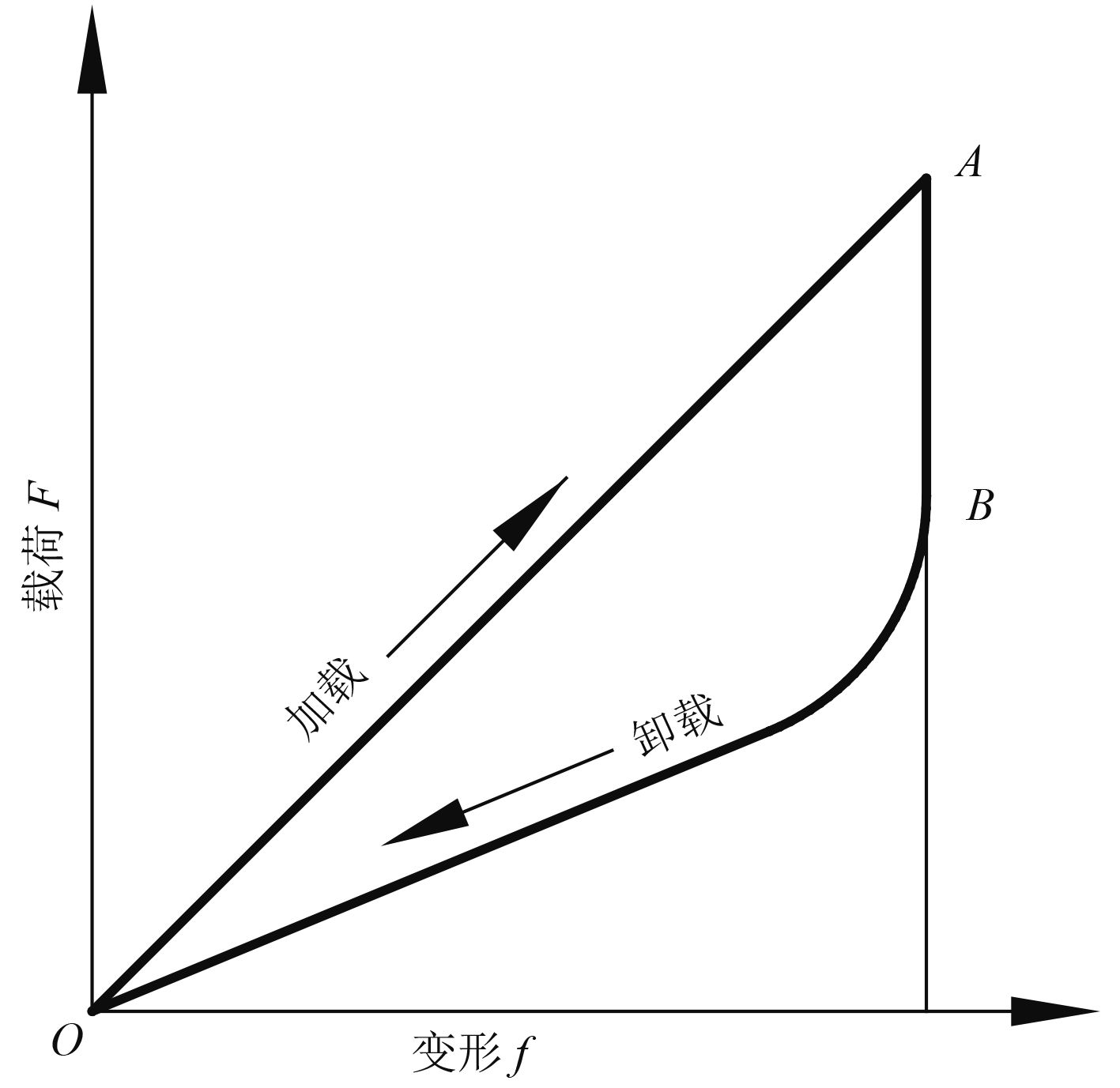图 1 环形弹簧力学性能曲线 Fig. 1 Annular spring mechanical property curve

ADAMS软件是功能强大的动力学仿真软件，在ADAMS中的弹簧刚度只能是固定值，不能设置不同的加载和卸载刚度直接模拟环形弹簧，本文用ADAMS中的一维作用力的表达式来模拟环形弹簧缓冲，勿需编写用户子程序

1 转管炮后坐缓冲受力分析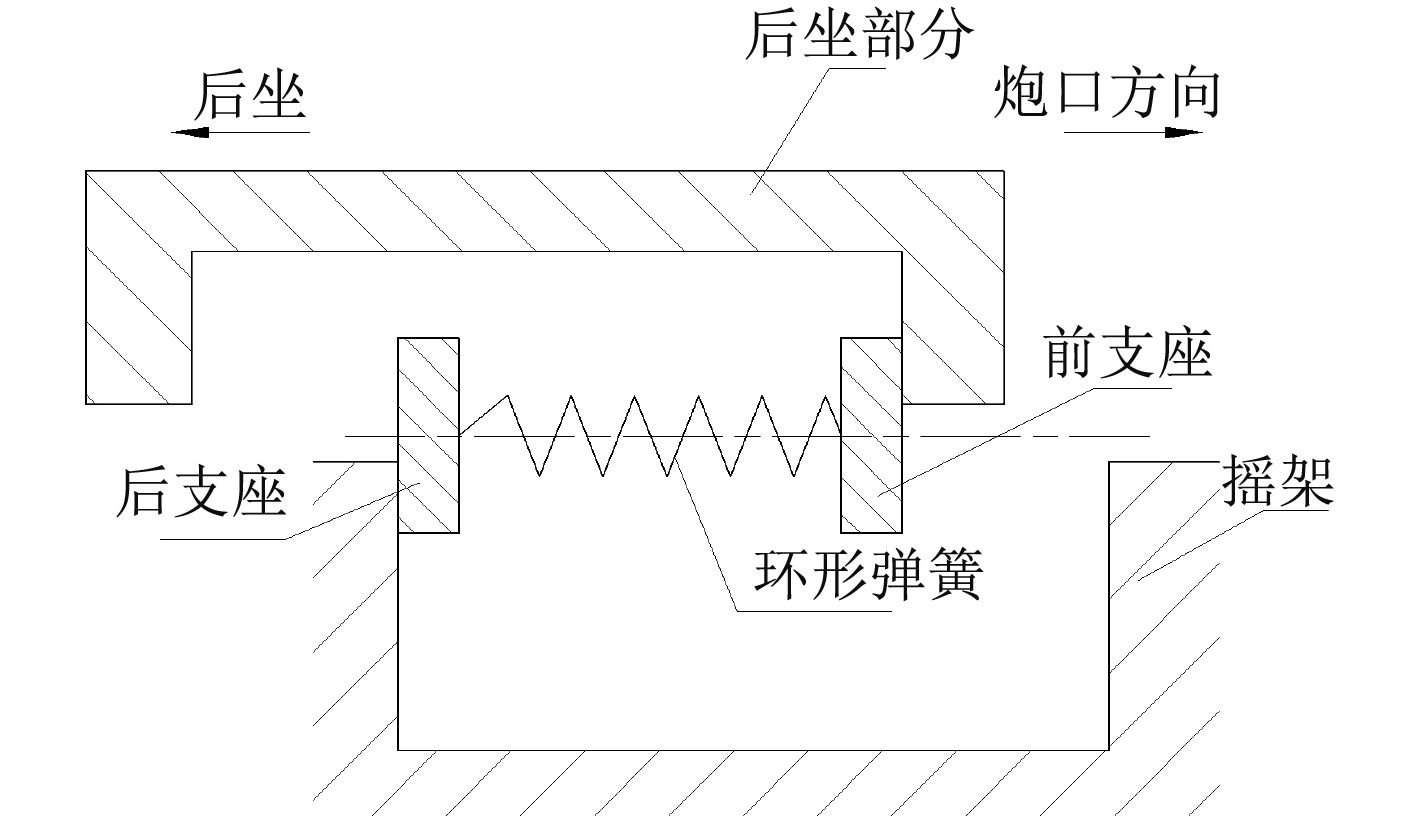图 2 后坐缓冲模型示意图 Fig. 2 Structure chart of the recoiling buffer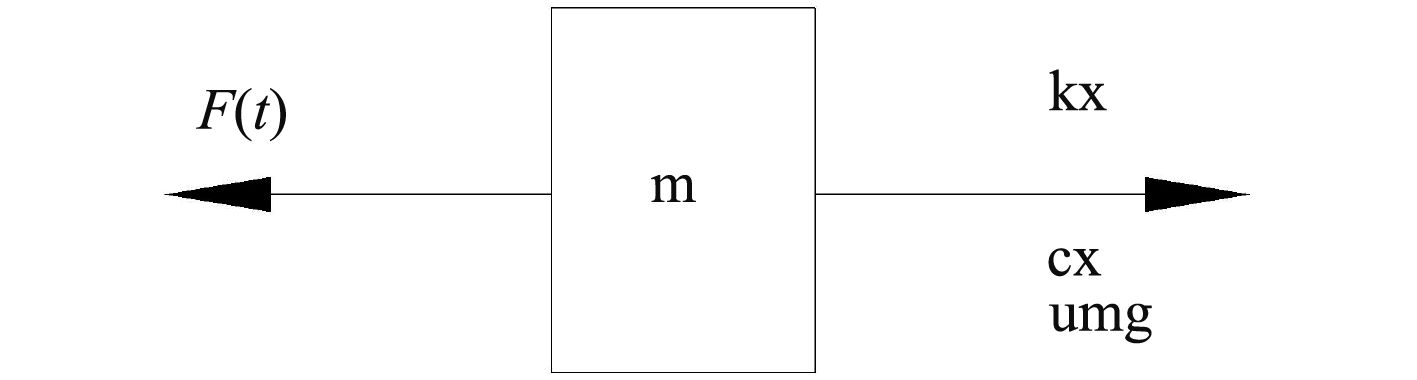图 3 后坐部分受力图 Fig. 3 force diagram of the Recoiling Buffer

 $F = m\ddot x = F(t) - k \cdot x - c \cdot \dot x - \mu \cdot m \cdot g\text{。}$

2 ADAMS下环形弹簧力学特性实现方法

1）当前支座处于后坐运动时：作用力=弹簧变形力+阻尼+摩擦力；

2）当前支座处于复进运动时：作用力=弹簧变形力×比例系数+阻尼×比例系数-摩擦力；

3）当后支座处于复进运动时：作用力=弹簧变形力+阻尼-摩擦力；

4）当后支座处于后坐运动时：作用力=弹簧变形力×比例系数+阻尼×比例系数+摩擦力。

${v_q}$ <0， $F = k \cdot x + c \cdot \dot x + \mu \cdot m \cdot g,$

${v_q}$ =0， $F = k \cdot x,$

${v_q}$ >0， $F = sc \cdot k \cdot x + sc \cdot c \cdot \dot x - \mu \cdot m \cdot g;$

${v_h}$ >0， $F = k \cdot x + c \cdot \dot x - \mu \cdot m \cdot g,$

${v_h}$ =0， $F = k \cdot x,$

${v_h}$ <0， $F = sc \cdot k \cdot x + sc \cdot c \cdot \dot x + \mu \cdot m \cdot g\text{。}$

2.1 按设计参数仿真

IF（后支座位移:

IF（前支座位移:

IF（前支座速度:

$k \cdot x + c \cdot \dot x + \mu \cdot m \cdot g$ , $k \cdot x$ , $sc \cdot k \cdot x + sc \cdot c \cdot \dot x - \mu \cdot m \cdot g$

）,

$k \cdot x$ , $k \cdot x$

）,

IF（前支座位移:

IF（前支座速度:

$k \cdot x + c \cdot \dot x + \mu \cdot m \cdot g$ , $k \cdot x$ , $sc \cdot k \cdot x + sc \cdot c \cdot \dot x - \mu \cdot m \cdot g$

）,

$k \cdot x$ , $k \cdot x$

）,

IF（后支座速度:

$sc \cdot k \cdot x + sc \cdot c \cdot \dot x + \mu \cdot m \cdot g,$ $k \cdot x,$ $k \cdot x + c \cdot \dot x - \mu \cdot m \cdot g$

2.2 用弹簧测试数据仿真

IF（后支座位移:

IF（前支座位移:

IF（前支座速度:

AKISPL（ $x$ , 0, SPLINE_1, 0）+ $c \cdot \dot x + \mu \cdot m \cdot g$ ,

AKISPL（ $x$ , 0, SPLINE_1, 0）,

AKISPL（ $x$ , 0, SPLINE_2, 0）+ $sc \cdot c \cdot \dot x - \mu \cdot m \cdot g$

）,

AKISPL（ $x$ , 0, SPLINE_1, 0）, AKISPL（ $x$ , 0, SPLINE_1, 0）

）,

IF（前支座位移:

IF（前支座速度:

AKISPL（ $x$ , 0, SPLINE_1, 0）+ $c \cdot \dot x + \mu \cdot m \cdot g$ ,

AKISPL（ $x$ , 0, SPLINE_1, 0）,

AKISPL（ $x$ , 0, SPLINE_2, 0）+ $sc \cdot c \cdot \dot x - \mu \cdot m \cdot g$

）,

AKISPL（ $x$ , 0, SPLINE_1, 0）, AKISPL（ $x$ , 0, SPLINE_1, 0）

）,

IF（后支座速度:

AKISPL（ $x$ , 0, SPLINE_2, 0）+ $c \cdot \dot x + \mu \cdot m \cdot g$ ,

AKISPL（ $x$ , 0, SPLINE_1, 0）,

AKISPL（ $x$ , 0, SPLINE_1, 0）+ $c \cdot \dot x - \mu \cdot m \cdot g$

3 仿真结果 3.1 依据设计参数仿真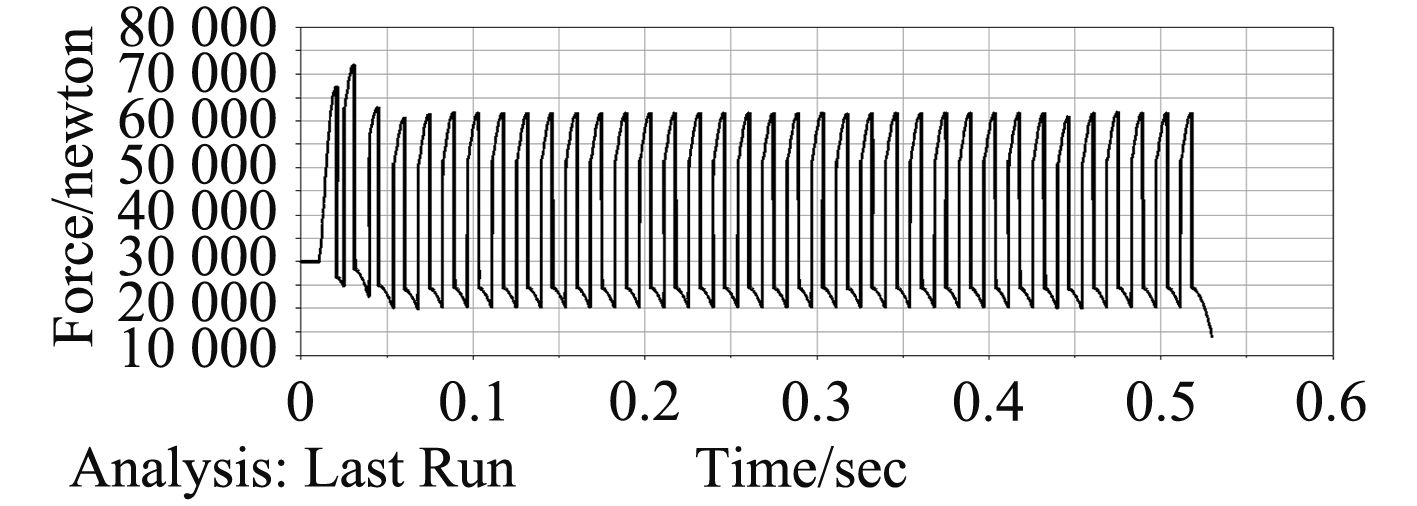图 4 弹簧力-时间曲线 Fig. 4 Spring force-time curve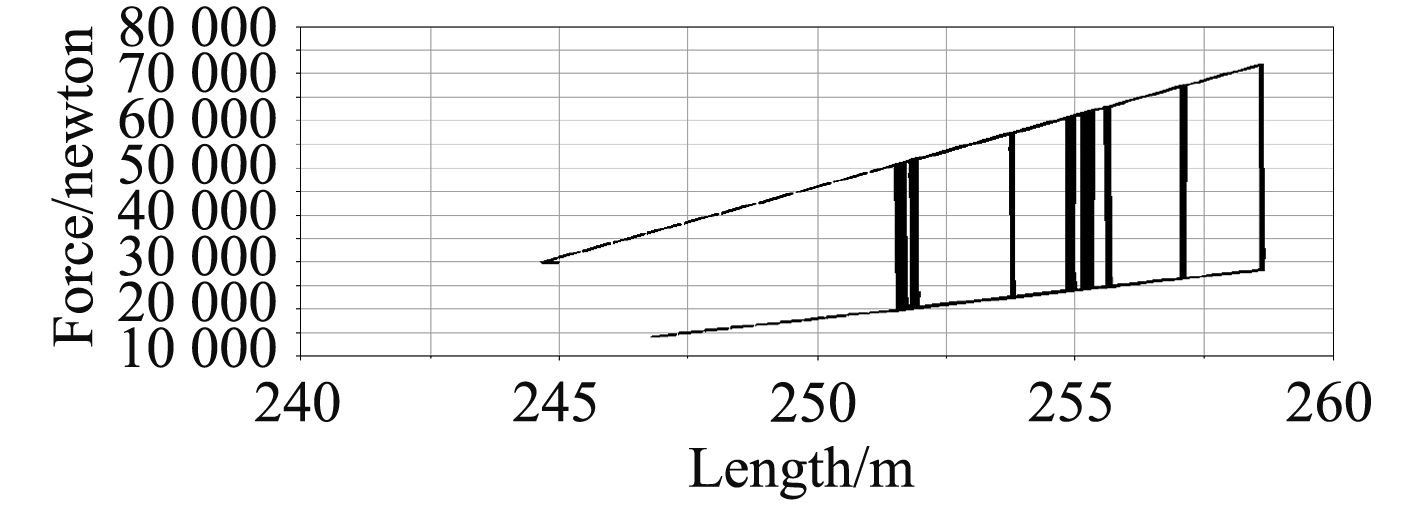图 5 弹簧力-位移曲线 Fig. 5 Spring force-displacement curve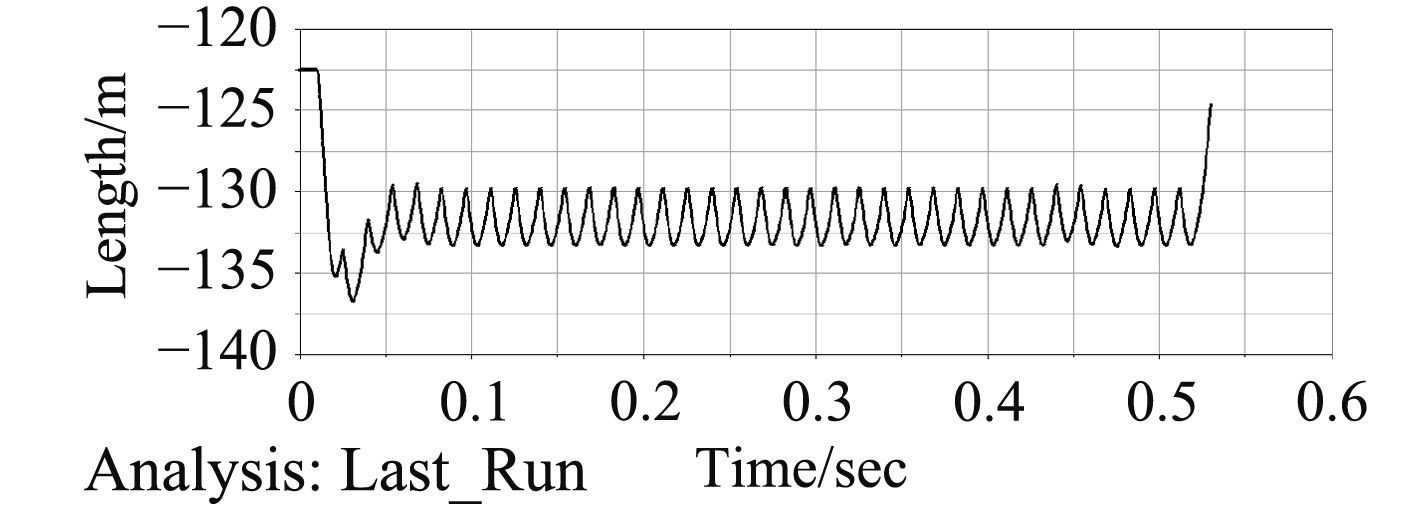图 6 自动机后坐位移-时间曲线 Fig. 6 Recoil displacement-time curve
3.2 以实际测试数据仿真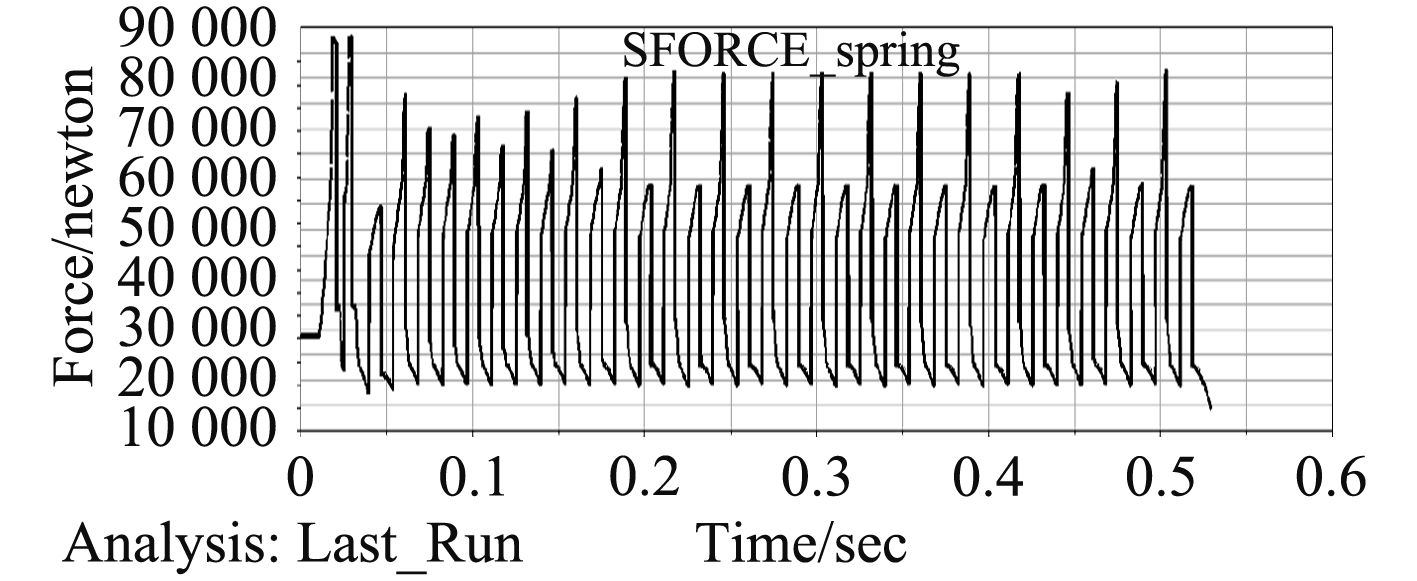图 7 弹簧力-时间曲线 Fig. 7 Spring force-time curve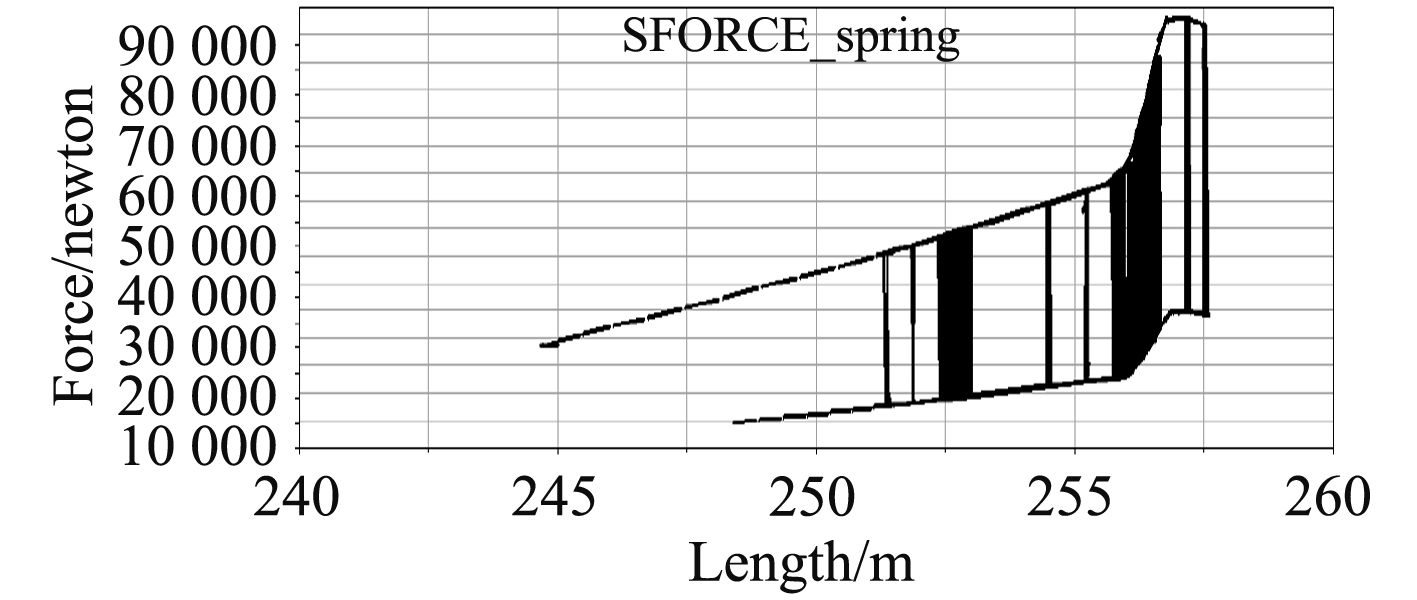图 8 弹簧力-位移曲线 Fig. 8 Spring force-displacement curve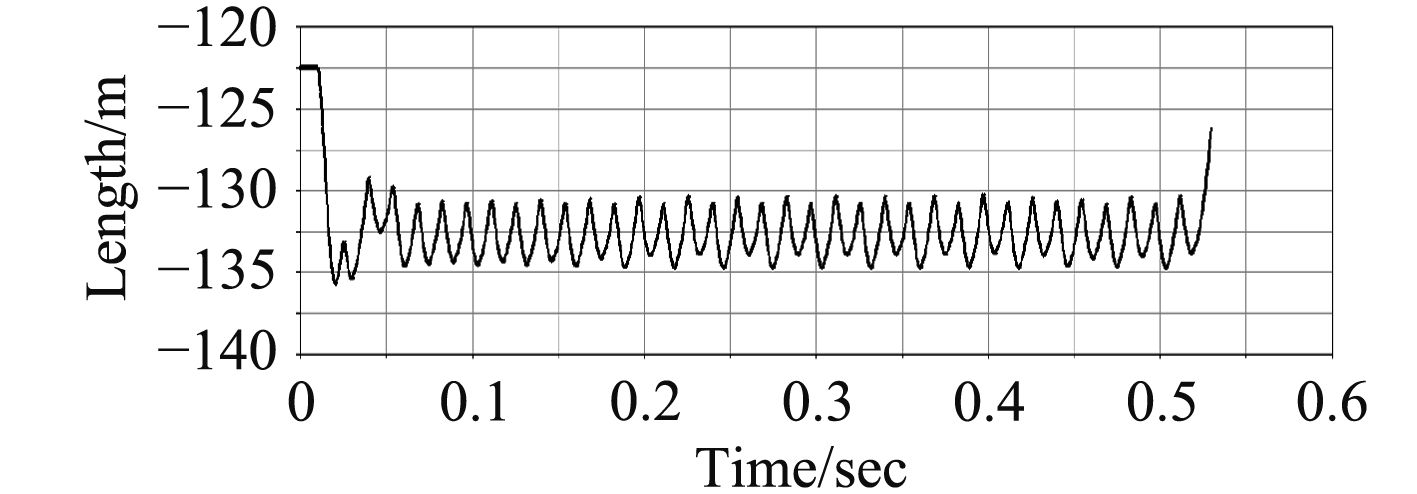图 9 后坐位移曲线（发射率4 200发/分） Fig. 9 Recoil displacement-time curve
4 与试验结果对比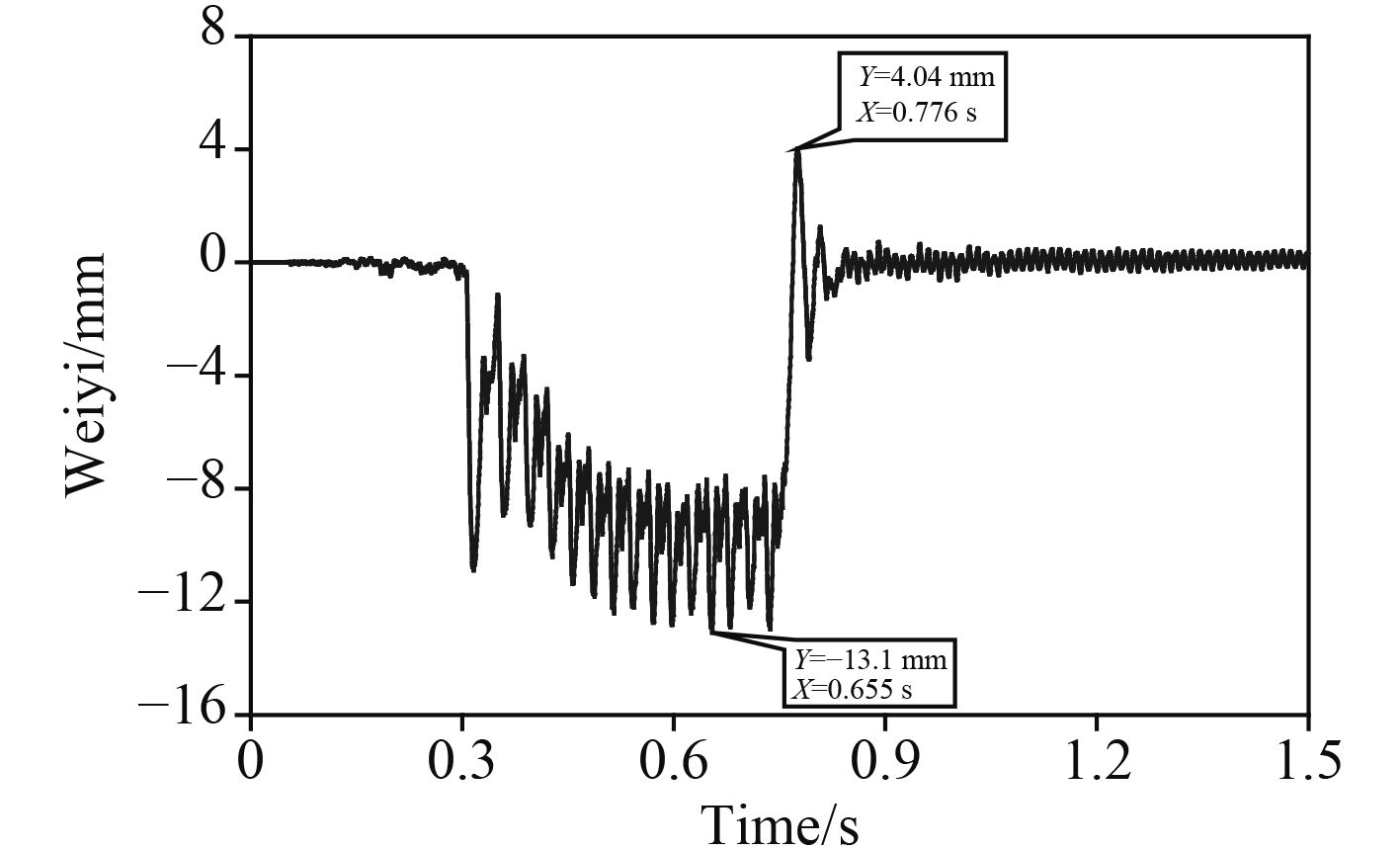图 10 实际射击测试后坐位移曲线（发射率4 200发/分） Fig. 10 Test dadta of recoil displacement-time curve
5 结　语

  成大先. 机械设计手册(第3卷)[M]. 第五版. 第11篇第9章环形弹簧. 北京: 化学工业出版社, 2014. CHENG Da-xian. Mechanical design handbook(3)[M]. Version 5. Chapter 9. Annular Spring. Beijing: Chemical Industry Press, 2014.  刘明敏, 张蔚峰, 朱延飞. 2种缓冲簧反后坐效能分析[J]. 舰船科学技术, 2014, 36(11): 157–161. LIU Ming-min, ZHANG Wei-feng, ZHU yan-fei. Efficiency analysis about two kinds of counter recoil equipment[J]. Ship Science and Technology, 2014, 36(11): 157–161.  周发明, 王宝元, 霍文妮, 等. 环形弹簧特性的RecurDyn子程序实现方法[J]. 火炮发射与控制学报, 2007(2): 21–23. ZHOU Fa-ming, WANG Bao-yuan, HUO Wen-ni, et al. Implementation of recurdyn subprogram with characteristics of ring spring[J]. Journal of Gun Launch and Control, 2007(2): 21–23.  贾长治, 杜秀菊, 刘广生, 等. 火炮冲击缓冲装置动态特性影响仿真分析与改进方法[J]. 机械工程学报, 2012, 48(19): 156–162. JIA Chang-zhi, DU Xiu-ju, LIU Guang-sheng, et al. Simulation and improvement of dynamic characteristics of impact buffering mechanism of guns based on virtual prototyping technology[J]. Journal of Mechanical Engineering, 2012, 48(19): 156–162.  申江森, 高跃飞, 徐凤军. 基于ADAMS的某突击炮发射动力学仿真[J]. 机械工程与自动化, 2015(2): 105–107. SHEN Jiang-sen, GAO Yue-fei, XU Feng-jun. ADAMS based dynamic simulation of assault gun firing[J]. Mechanical Engineering & Automation, 2015(2): 105–107. http://doi.wanfangdata.com.cn/10.3969/j.issn.1672-6413.2015.02.042